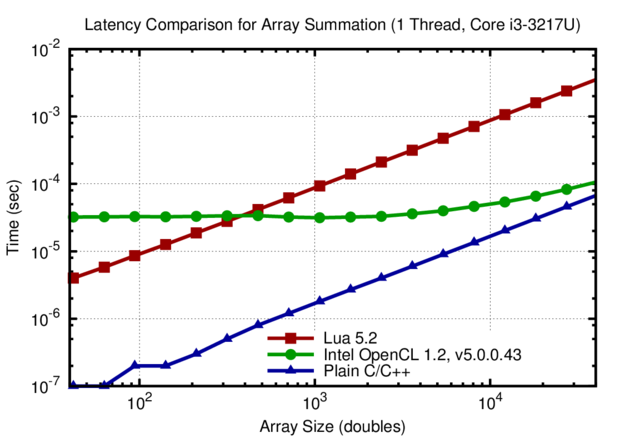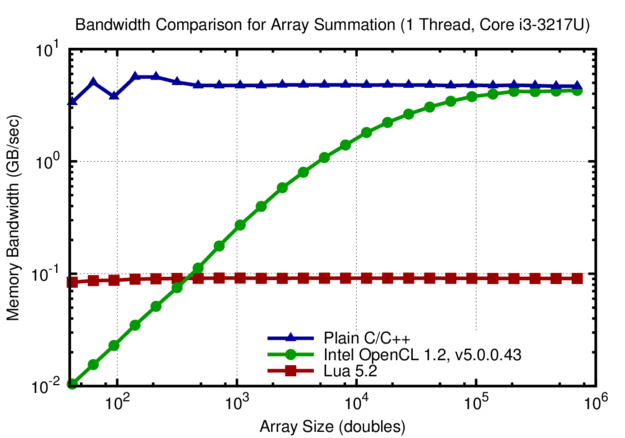# Latency Comparison of Lua, OpenCL, and native C/C++

Just-in-time compilation is an appealing technique for producing optimized code at run time rather than at compile time. In an earlier post I was already looking into the just-in-time compilation overhead of various OpenCL SDKs. This blog post looks into the cost of launching OpenCL kernels on the CPU and compares with the cost of calling a plain C/C++ function through a function pointer, and with the cost of calling a precompiled Lua script.

Small latency is essential for good composability of software. For example, sorting routines such as `std::sort()` in the C++ Standard Template Library take an optional comparison functor for comparing two elements in the array. The general assumption for such an application programming interface (API) is that the comparison functor can be called at low cost compared to the actual operations. With tricks such as template metaprogramming, these overheads can indeed be kept negligible within the C++ world. If, on the other hand, each call to the comparison functor takes several thousand processor cycles of processing overhead, performance will be disastrous.

The example we consider here is the summation of all elements of an array of double precision floating point values. Single-threaded execution is considered for the sake of clarity. A possible implementation in C (or C++) for summing an array `x` with `N` elements is as follows:

```// C function for summing all elements of an array
double compute_sum(double *x, size_t N) {
double sum = 0;
for (size_t i=0; i<N; ++i)
sum += x[i];
return sum;
}
```

Since the function `compute_sum` is compiled through the standard C or C++ compiler, there is no overhead other than the function call itself. Thus, the execution time scales with the number of elements `N`. This means that the cost of the function call itself (i.e. placing arguments on the stack, etc.) are amortized by the work in the for-loop already for small values of `N`.

Let us now turn to the OpenCL implementation for achieving the same. The OpenCL kernel for a single thread is almost identical to the C/C++ example above:

```// OpenCL kernel for summing all elements of an array
__kernel void compute_sum(__global const double * x,
__global       double * sum,
unsigned int N) {
double s = 0;
for (unsigned int i = 0; i < N; ++i)
s += x[i];
*sum = s;
};
```

Calling the OpenCL kernel after the jit-compilation is more involved than a simple function call: We have to specify the three arguments via clSetKernelArg(), then enqueue the kernel via clEnqueueTask() or clEnqueueNDRangeKernel(), and finally retrieve the result via clEnqueueReadBuffer(). Each of these calls requires a certain amount of error checking, so the kernel launch overhead is necessarily higher than a single function call.

For comparison I also added a Lua script to the benchmark. The reason for choosing Lua is that a Lua script is just-in-time-compiled at run time and callable from a C API, just like OpenCL kernels. In contrast to OpenCL, the script is not compiled to native machine code, so some additional overhead for executing each operation in the Lua function is expected. The Lua code for computing the sum of all entries of an array is again very similar to the previous code snippets:

```-- Lua script for summing all elements of an array
function compute_sum(bar, N)
x = 0
for i = 1, N do
x = x + bar[i]
end
return x
end
```

The Lua function is called from C/C++ by pushing the array elements into a table object on the virtual stack. Since the process of calling an OpenCL kernel and a Lua function as well as retrieving the result are similar, a-priori one expects similar overhead.

## Results

The code in the benchmark repository supplementing this blog post is available on GitHub and was run on a laptop equipped with an Intel i3-3217U CPU clocked at 1.8 GHz. Results are expected to be similar across different hardware. The numbers are median values of 10 runs, where each run represents the average time per run over 10 runs.

The execution time for different array sizes are as follows:Comparison of execution times for summing the values of arrays with different length. The execution time for the Lua and plain C implementations scale linearly down to array sizes below 100 entries. In contrast, the overhead with the Intel OpenCL SDK is 40 microseconds and leads to constant execution time for arrays with up to 10k elements.

The large difference between OpenCL and Lua is surprising: The latency of calling a Lua function is by about an order of magnitude smaller than calling an OpenCL function. This is surprising given that both approaches have similar semantics for launching a kernel. A possible explanation is the extra overhead for assigning the work to a (new?) thread in OpenCL, whereas Lua uses the main thread for the kernel.

Translating the results into effective memory bandwidth results in the following plot:Comparison of effective memory bandwidth for summing the values of arrays with different length. The high latency of the Intel OpenCL SDK causes a drop increase in effective memory bandwidth below 10k array elements. In contrast, the effective memory bandwidth is constant for the Lua script and the plain C/C++ implementation.

The implementation in Lua is by two orders of magnitudes slower than the compiled C/C++ implementation. The OpenCL implementation reaches the same effective memory bandwidth for large arrays, but suffers from the kernel launch overhead for arrays with less than 10k elements.

## Conclusion

It was surprising to see the Lua implementation outperform the OpenCL implementation by up to an order of magnitude for array sizes below 100. This suggests that there is significant optimization potential in the Intel OpenCL SDK. After all, the OpenCL kernel is compiled to x86 code and thus the OpenCL kernel launch ultimately boils down to just a function call. One may wonder why it takes the Intel OpenCL SDK longer to launch a native function than it takes the OpenCL SDKs from NVIDIA and AMD to launch a GPU kernel (including PCI-Express communication!). Only for large data the OpenCL kernel matches the performance of the plain C/C++ implementation.

In summary, the kernel launch latency problems of OpenCL on CPUs is one of the big show-stoppers for a more wide-spread adoption of OpenCL for processing on the CPU. A possible remedy is to offer additional interfaces in OpenCL which allow for data processing by the main thread, thus circumventing thread synchronization overheads.

Resources: Benchmark code repository on GitHub

This blog post is for calendar week 11 of my weekly blogging series for 2016.